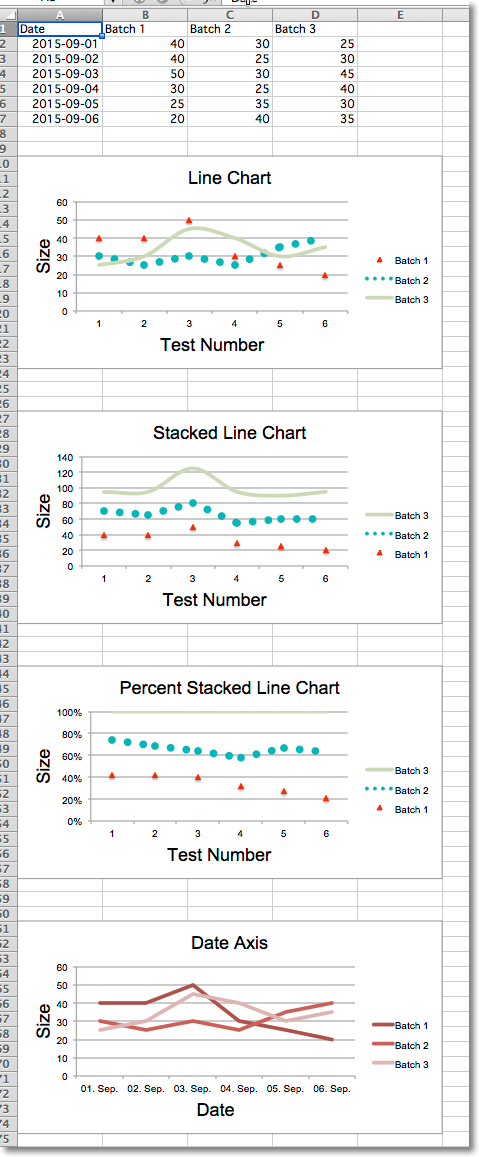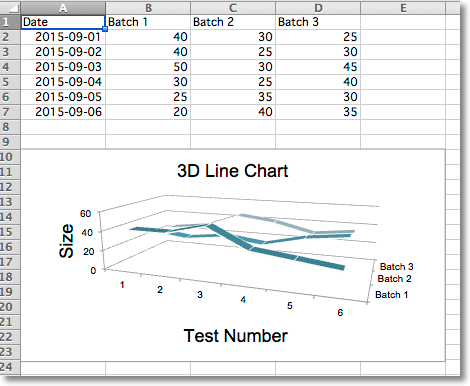# Line Charts¶

## Line Charts¶

Line charts allow data to be plotted against a fixed axis. They are similar to scatter charts, the main difference is that with line charts each data series is plotted against the same values. Different kinds of axes can be used for the secondary axes.

Similar to bar charts there are three kinds of line charts: standard, stacked and percentStacked.

```from datetime import date

from openpyxl import Workbook
from openpyxl.chart import (
LineChart,
Reference,
)
from openpyxl.chart.axis import DateAxis

wb = Workbook()
ws = wb.active

rows = [
['Date', 'Batch 1', 'Batch 2', 'Batch 3'],
[date(2015,9, 1), 40, 30, 25],
[date(2015,9, 2), 40, 25, 30],
[date(2015,9, 3), 50, 30, 45],
[date(2015,9, 4), 30, 25, 40],
[date(2015,9, 5), 25, 35, 30],
[date(2015,9, 6), 20, 40, 35],
]

for row in rows:
ws.append(row)

c1 = LineChart()
c1.title = "Line Chart"
c1.style = 13
c1.y_axis.title = 'Size'
c1.x_axis.title = 'Test Number'

data = Reference(ws, min_col=2, min_row=1, max_col=4, max_row=7)

# Style the lines
s1 = c1.series
s1.marker.symbol = "triangle"
s1.marker.graphicalProperties.solidFill = "FF0000" # Marker filling
s1.marker.graphicalProperties.line.solidFill = "FF0000" # Marker outline

s1.graphicalProperties.line.noFill = True

s2 = c1.series
s2.graphicalProperties.line.solidFill = "00AAAA"
s2.graphicalProperties.line.dashStyle = "sysDot"
s2.graphicalProperties.line.width = 100050 # width in EMUs

s2 = c1.series
s2.smooth = True # Make the line smooth

from copy import deepcopy
stacked = deepcopy(c1)
stacked.grouping = "stacked"
stacked.title = "Stacked Line Chart"

percent_stacked = deepcopy(c1)
percent_stacked.grouping = "percentStacked"
percent_stacked.title = "Percent Stacked Line Chart"

# Chart with date axis
c2 = LineChart()
c2.title = "Date Axis"
c2.style = 12
c2.y_axis.title = "Size"
c2.y_axis.crossAx = 500
c2.x_axis = DateAxis(crossAx=100)
c2.x_axis.number_format = 'd-mmm'
c2.x_axis.majorTimeUnit = "days"
c2.x_axis.title = "Date"

dates = Reference(ws, min_col=1, min_row=2, max_row=7)
c2.set_categories(dates)

wb.save("line.xlsx")
```## 3D Line Charts¶

In 3D line charts the third axis is the same as the legend for the series.

```from datetime import date

from openpyxl import Workbook
from openpyxl.chart import (
LineChart3D,
Reference,
)
from openpyxl.chart.axis import DateAxis

wb = Workbook()
ws = wb.active

rows = [
['Date', 'Batch 1', 'Batch 2', 'Batch 3'],
[date(2015,9, 1), 40, 30, 25],
[date(2015,9, 2), 40, 25, 30],
[date(2015,9, 3), 50, 30, 45],
[date(2015,9, 4), 30, 25, 40],
[date(2015,9, 5), 25, 35, 30],
[date(2015,9, 6), 20, 40, 35],
]

for row in rows:
ws.append(row)

c1 = LineChart3D()
c1.title = "3D Line Chart"
c1.legend = None
c1.style = 15
c1.y_axis.title = 'Size'
c1.x_axis.title = 'Test Number'

data = Reference(ws, min_col=2, min_row=1, max_col=4, max_row=7)